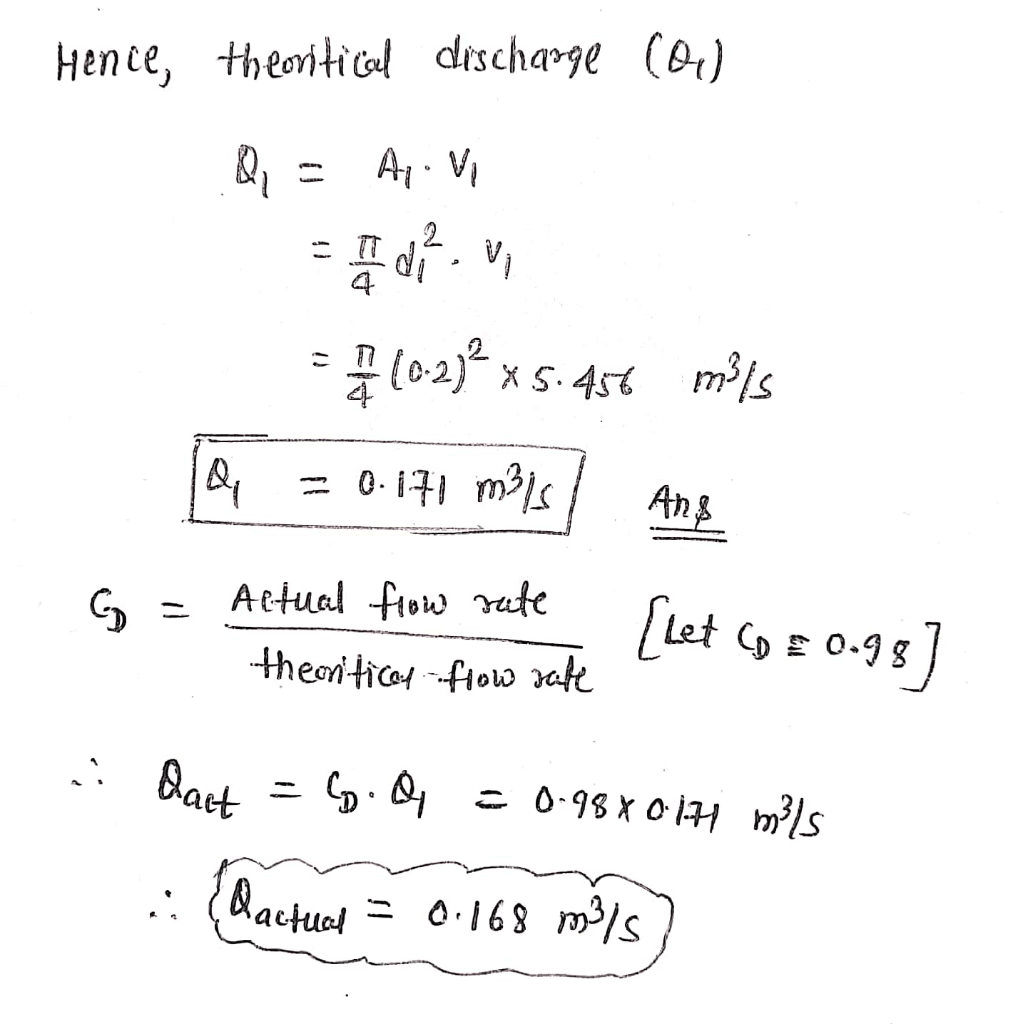# A horizontal orifice meter is used to measure the flow rate of water through the piping system of 20 cm I.D, where the diameter of throat in the meter is d2 10 cm. The pressure at inlet is 17.658 N/cm2 gauge and the vacuum pressure of 35 cm Hg at throat. Find the discharge of water.

Question-AnswerCategory: Fluid MechanicsA horizontal orifice meter is used to measure the flow rate of water through the piping system of 20 cm I.D, where the diameter of throat in the meter is d2 10 cm. The pressure at inlet is 17.658 N/cm2 gauge and the vacuum pressure of 35 cm Hg at throat. Find the discharge of water.

A horizontal orifice meter is used to measure the flow rate of water through the piping system of 20 cm I.D, where the diameter of throat in the meter is d2 10 cm. The pressure at inlet is 17.658 N/cm2 gauge and the vacuum pressure of 35 cm Hg at throat. Find the discharge of water.